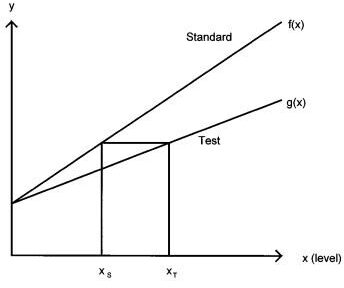# Slope Ratio Calculator### How to calculate statistics for slope ratio assays

The Slope Ratio Assay Excel workbook was written to help clarify and automate the procedures for calculating the standard errors and fiducial limits for slope ratio assays.  The procedures are based on the chapter “Statistical Evaluation of Bioavailability Assays” by Littell, Lewis and Henry in the book BIOAVAILABILITY OF NUTRIENTS FOR ANIMALS:  AMINO ACIDS, MINERALS AND VITAMINS, Copyright 1995 by Academic Press, Inc.

The Excel workbook “Slope Ratio Assay” contains example SAS code following the Littell et al. (1995) procedures.  Below is the SAS output from that example.  Transfer the data highlighted in green from the pages below to the Excel workbook to make the calculations.

Gene Pesti